##### Page tree
Go to start of banner

# TK514 Interrupter# Teori

TBD

## Virkemåte

The interrupter generates the signal which drives the resonant circuit (coil rig) at its resonant frequency f0. As long as the input signal X2 is high the output produces a square wave with fundamental frequency f0. It does this by means of a positive feedback loop. The feedback signal X8 is retrieved with a sensing transformer around the output wire from the power amplifier (section 2.4), before being clamped, rectified, and schmidt triggered. This results in a cleaned up normalized representation of X8, lets call this signal X80. The flanks of X80 represents when the output current passes zero (this is when we want to switch the polarity of the output X5). X80 is fed to the output via gates controlled by a latch. X5_B is inverted in relation to X5_A (for push-pull operation). This circuit is shown in fig. 2.5, U1A is the latch witch is central to the operation of the interrupter. It has Four inputs SD, CP, D, and RD, wich are ’Set Data’ (active low), ’Clock Pulse’, ’Data’, and ’Reset Data’ (active low) respectively. And two outputs; Q wich is the normal output, and Q inverted wich is the inverted of Q at all times, Q inverted is unused in this circuit.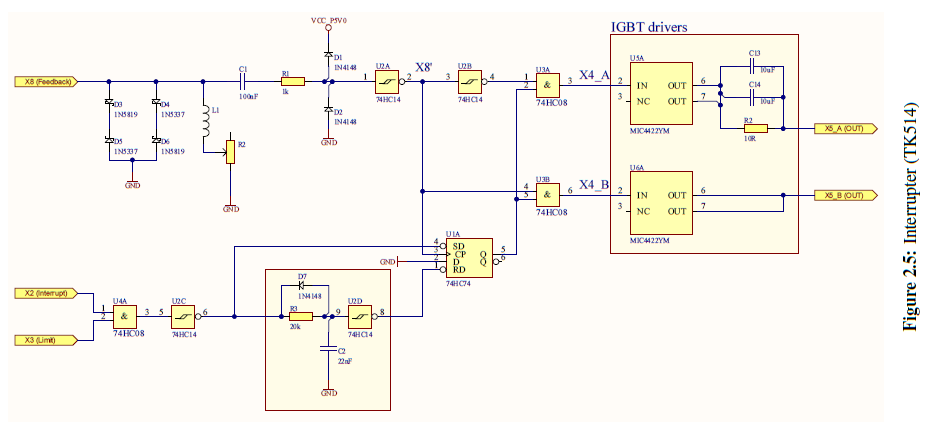Initially no current is flowing in the resonant circuit therefore no voltage is present on X8, but because of C1 the the input of U2A is undefined. Let us look at the case of the output of U2A being high and the input X2 being low. Then the initial values of the signals are as shown in fig. 2.6, SD is high (inactive) CP is high, D is low, RD is low (active), Q is low, X4_A and X4_B is low.When X2 goes high the Set Data (SD) input of the latch U1A goes low and is activated, Reset Data RD goes high (inactive), and thus the output Q goes high. This enables the and gates U3A and U3B and allows X8’ to pass through, X4_B goes high and X4_A remains low, this causes a step response in the resonant circuit. The feedback transformer is oriented such that this current direction gives a negative voltage on X8 and thus still low signal on the input of U2A. When the current direction changes the signal on the input of U2A goes from low to high and X4_A goes high and X4_B goes low. This reverses the voltage on the resonant circuit in phase with the step response and triggers an additional step response in phase with the already ongoing one. This cycle continues untilX2 goes low. When X2 goes low SD goes low (inactive), but Q is still high until the next negative flank on X8 (inverted through U2A). When D (wich is strapped low) is clocked through the latch, Q goes low and both X4_A and X4_B goes low. And no further energy is supplied to the resonant circuit and the step response completes. In the case of the initial value of the output of U2A being low X4_A would go high first instead of X4_B and X8 would go high right away. Prompting X4_A and X4_B to invert immediately and switch the output while the current is not zero. Then the rest of the events happen as explained above. This immediate inverting of X4 may be unfortunate and a pull down resistor should be added at the input of U2A. The resulting signal on the output X7 is then Pulse Density Modulated (PDM). Phase Lead The function of L1 and R2 is to introduce a phase lead on the voltage of X8 in relation of the current on X8. The purpose of this is to compensate for propagation delays in the circuit and for switching delays in the transistors in the power amplifier (section 2.4). As the circuit is inductive, the voltage will lead the current. By adjusting the value of R2 the relation between the inductance and resistance is changed and thus the phase angle is changed. Thus the time between when the voltage crosses zero and the current crosses zero can be adjusted. This time should theoretically be equal to the time it takes the feedback signal X8 to propagate through the logic and for the transistors (IGBTs) in the power amplifier to turn on or off. So that we will switch when the current in the resonant circuit is zero (Zero current switching). This is to reduce energy lost from the resonant circuit and to minimize power burned in the transistors (when switching). From the datasheets we have the propagation delays tpd for the different devices in the propagation path shown in table 2.1. The there are two propagation paths one path contains one schmidt trigger (74HC14) more than the other, also the propagation delay in the mosfet drivers (MIC4422YM) differs for rising and falling outputs. The average propagation delay tpd from X8 on the input of U2A to X5 on the output of the mosfet drivers is then given by eq. (2.1) and results in tpd = 58 ns typical and 137,5 ns maximum.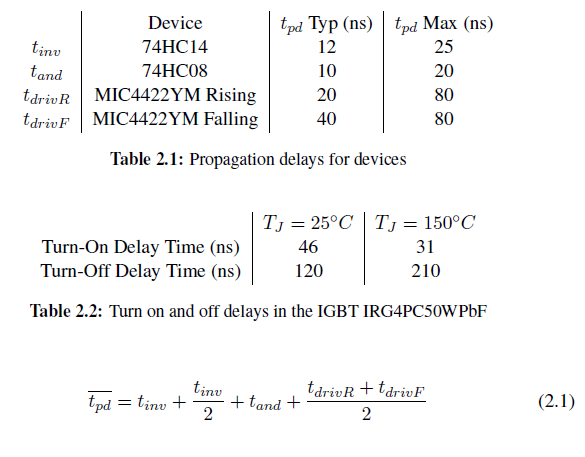In addition the delays from hysteresis in U2A th and switching delays in the transistors in the power amplifier tsw should be added to the desired phase lead td. Resulting in the desired phase lead td being given by eq. (2.2).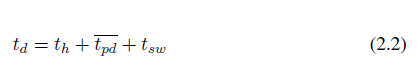The feedback signal X8 should have sufficiently high voltage so that delays from hysteresis th in U2A is neglible. Delays in the IGBT is read from the datasheet and presented in table 2.2. If we add the delay at 25 C to the nominal td and the delay at 150C to the maximum td, we get a td of 141 ns nominal and 258 ns maximum. Since there are no other resistances in the circuit than R2, as R1 is in series with both a capacitor and the input of the logic gate U2A and can be considered close to infinite in relation to R2, the phase angle is given by eq. (2.3).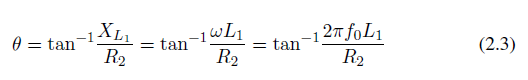And thus the desired phase lead is given by eq. (2.4), and the relation between L1 and R2 is given by eq. (2.5).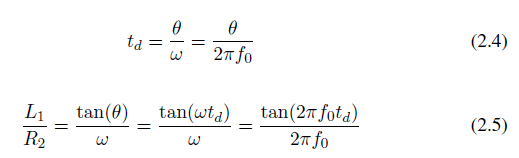Given f0 = 110kHz and desired td = 141 ns nominal and 258 ns maximum we get L R = 1; 4 10􀀀7 s nominal and L R = 2; 6 10􀀀7 s maximum. The total magnitude jZLj of the impedance ZL of L1 and R2 should give a sufficiently high voltage UX8 so that the delay due to hysteresis in U2A is negligible, but not too high voltage for the zener diodes D3-D6 to handle. The equation for jZLj is shown in eq. (2.6).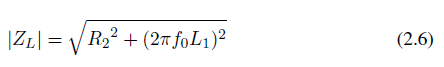The relation between the peak voltage jUX8j over R2 and L1 is shown in eq. (2.7) assuming X8 is sinusiodal.### Reset network

The function of the network connected to the reset (RD) of the latch (U1A) is to reset the latch after a delay in the case that a zero crossing is not detected on X8 after X2 goes low. Note the inverting schmidth triggers U2C and U2D on both sides of the network. When X2 goes high the input of U2D goes low immediately due to the capacitor C2 being discharged through D7, but when X2 goes low the capacitor C2 will be charged through R3 and there will be a delay before the latch is reset. The time constant of R3 C2 is = 440 10􀀀6s, the positive going threshold voltage of the inverting schmitt trigger (74HC14) is T+ = 2; 5V Wich is half of the supply voltage VCC_P5V0. We know that a capacitor is charged to 0; 5 VCC (where VCC is the applied voltage) after 0; 7 , thus the filter R3 C2 together with U2D introduces a delay of 0; 7 = 308s, or if we have a resonance frequency f0 of 110 kHz a delay of about 3,4 periods T = 1 f0 . If the synchronous shutdown does not work properly this filter should prevent or reduce noise from the interrupter not shutting down properly between each pulse on the input signal X2. This can also reduce the spark length if the spark is prevented from unintentionally continue longer than intended.

### Input clamping and protection

D3-D6 are protection diodes which clamp the feedback signal to safe voltages. The network L1 and R2 introduces a tunable phase lead on the voltage. C1 and R1 is a filter to remove noise. D1 and D2 clamps the voltage to 0-5V.

### IGBT Drivers

U5A and U6A are transistor drivers which amplify X4 and step up the voltage from 5V to 18V

# Spec

Interruptindikator for alle slemme interrupts

Separat indikator for signalinterrupt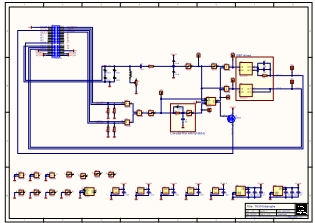# Versjoner

## V0.0 (2009)

### Changelog

1. Laget av Dewald De Bruyn

1. Ingen

## V0.1 (2014)

### Changelog

1. Kokt fra 2009 design

1. Ingen

## V1.0

Release: 2017-03-16
Antall: 10

### Changelog

1. Bakplanifisert
2. Lagt til testpunkter
3. Lagt til (footprint for) potmeter og spole R4, L1 for å kunne justere phase lead

### Errata

1. Mangler thermals på jordpinner.
2. C10 og C11 kan "krympes" til 0805
3. C15 kan krympes til 0603
4. D1, D2, D7 kan krympes til 0603

# Produserte kort

SNVMontertFungerer

PlasseringKommentar
?0.02009 Driver
?0.12014 Driver
?0.1Marintesla
011.0TK500 SN:01Phase lead ikke justert, muligens ikke montert
021.0TK500 SN:02Ikke testet
031.0041.0ØysteinIkke testet
051.0

061.0

071.0

081.0

091.0

101.0

• No labels